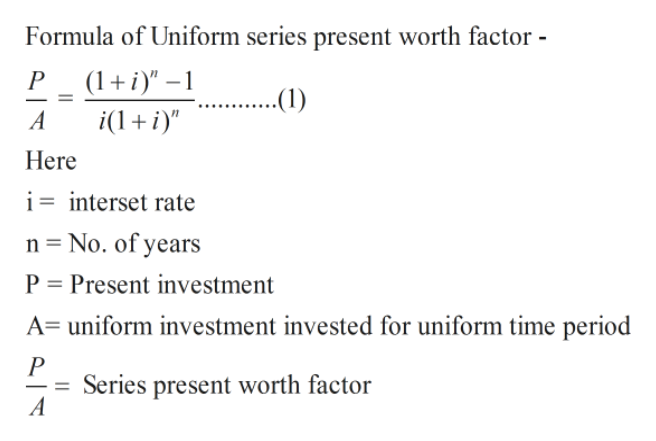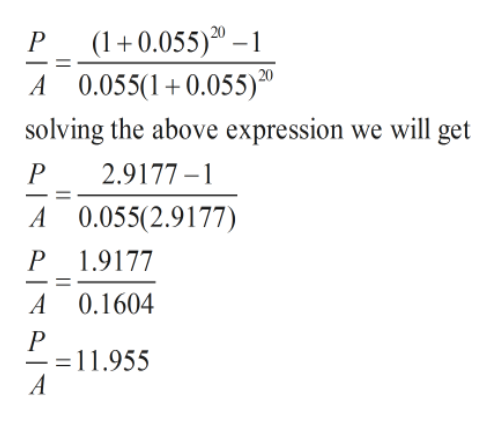# 3. What is the Uniform Series Present Worth factor for an interest rate of 5.5% over 20 years?

Question
18 views

3. What is the Uniform Series Present Worth factor for an interest rate of 5.5% over 20 years?

check_circle

Step 1

Solution - From the question

Uniform series - It is a series of uniform payments which are   uniform in  amount are   uniform spaced.

Uniform worth factor - Uniform worth factor used to calculate unifor payment  for  uniform amount of time .

Given in the question

Interset rate (i) = 5.5%

No. of Years (n) = 20

We have to calulate

Uniform Series present worth payment  = ?help_outlineImage TranscriptioncloseFormula of Uniform series present worth factor P ( ..(1) A i(1 Here i= interset rate n No. of years P Present investment A uniform investment invested for uniform time period P Series present worth factor A _ fullscreen
Step 2

In eqn.(1)  putting the va...help_outlineImage Transcriptionclose(1+0.055)30-1 A 0.055(10.055)20 P solving the above expression we will get P 2.9177- A 0.055(2.9177) P 1.9177 A 0.1604 11.955 A fullscreen

### Want to see the full answer?

See Solution

#### Want to see this answer and more?

Solutions are written by subject experts who are available 24/7. Questions are typically answered within 1 hour.*

See Solution
*Response times may vary by subject and question.
Tagged in